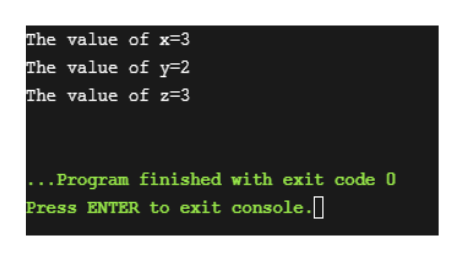# x = 2, y =1, z =3, what is the value of x, y, z after executing the following codes?switch(x){case 0: x =2; y =3;case 1: x =3; break;default: x=3; y=2;}

Question
318 views
x = 2, y =1, z =3, what is the value of x, y, z after executing the following codes?
switch(x)
{
case 0: x =2; y =3;
case 1: x =3; break;
default: x=3; y=2;
}
check_circle

Step 1

Code (in java)-

class Main

{

public static void main (String [] args)

{

int x = 2, y =1, z =3;//initializing the variables

switch(x)

{

case 0: x =2; y =3;

case 1: x =3; break;

default: x=3; y=2;

}

//Displaying the final values of x, y and z

System.out.println("The value of x="+x);

System.out.println("The value of y="+y);

System.out.println("The value of z="+z);

}

}

Step 2

Output-help_outlineImage TranscriptioncloseThe value of x=3 The value of y=2 The value of z=3_ . Program finished with exit code 0 Press ENTER to exit console.0 fullscreen
Step 3

Explanation-

Initialize the variables x, y and z as per given in the question.

Checking each case of switch statement.

As the value of x is 2, so none of the cases...

### Want to see the full answer?

See Solution

#### Want to see this answer and more?

Solutions are written by subject experts who are available 24/7. Questions are typically answered within 1 hour.*

See Solution
*Response times may vary by subject and question.
Tagged in